# 闵氏空间与常曲率空间的几何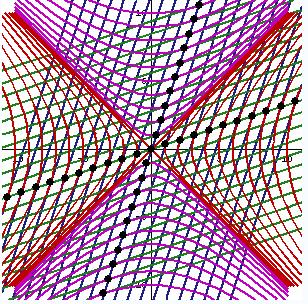## 特色内容

• 双曲函数与双曲角
• 闵氏空间中的物理学
• 德西特与反德西特时空几何
• 内蕴几何与内禀曲率
• 黎曼曲率张量的几何意义

$s^2=(x_2-x_1)^2-(y_2-y_1)^2$

$x^2-y^2=R^2$

## 双曲函数与双曲角

$$\mathrm{sinh}(\alpha)={e^{x}-e^{-x}\over 2}$$ $$\mathrm{cosh}(\alpha)={e^{x}+e^{-x}\over 2}$$这些指数函数跟正弦、余弦、双曲线有什么关系呢？其实上面这种定义没有体现双曲线的本质，下面介绍关于双曲函数的几何定义。三角函数可以定义在单位圆内，类似地，双曲函数也可以定义在“单位”双曲线内(微积分可算出它们就是上面的指数表达式)。我们知道在最自然的弧度单位制下，三角函数的自变量是圆心角，其值等于它所对应的弧长，也等于它夹出来的扇形面积的一半。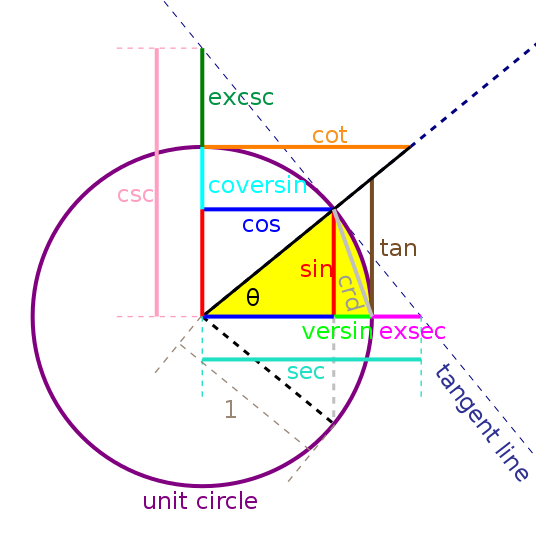#### 双曲角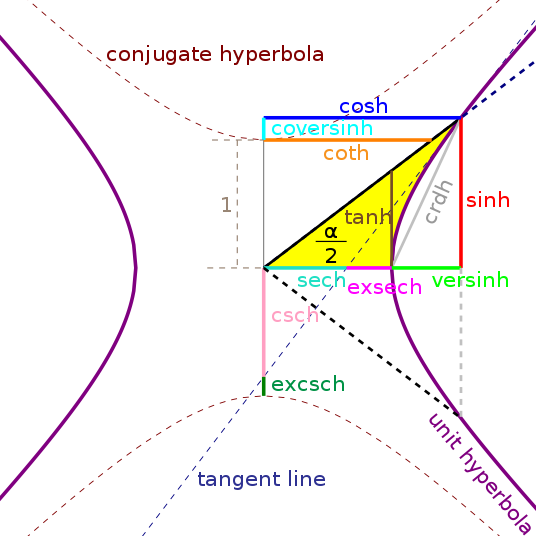### 闵可夫斯基世界中的“双曲旋转”

1. 直角坐标转极坐标：$\alpha=\mathrm{artanh}(y/x)$，$r=\sqrt{x^2-y^2}$；
2. 旋转双曲角$\beta$：$\alpha’=\alpha+\beta=\mathrm{artanh}(y/x)+\beta$，$r’=r=\sqrt{x^2-y^2}$；
3. 转换回直角坐标：$x’=r’\mathrm{cosh}(\mathrm{artanh}(y/x)+\beta)$，$y’=r’\mathrm{sinh}(\mathrm{artanh}(y/x)+\beta)$；

$$\mathrm{sinh}(A+B)=\mathrm{sinh}(A)\mathrm{cosh}(B)+\mathrm{cosh}(A)\mathrm{sinh}(B)$$ $$\mathrm{cosh}(A+B)=\mathrm{cosh}(A)\mathrm{cosh}(B)+\mathrm{sinh}(A)\mathrm{sinh}(B)$$ 化简得到：$$x’=r’(\mathrm{cosh}(\mathrm{artanh}(y/x))\mathrm{cosh}(\beta)+\mathrm{sinh}(\mathrm{artanh}(y/x))\mathrm{sinh}(\beta))$$

### 闵氏世界的奇特方向## 我们都生活在闵可夫斯基世界

##### 二维时空

1. 相对论的不同速度的变换叫洛伦兹变换，也就是我们刚才说的双曲旋转
翻译：直接看下面这张动图感受一下：以运动的物体为参考系，当物体加减速时就需要旋转时间轴使得这个参考系下的y轴始终与世界线相切。注意动画中叠加了沿曲线的平移和双曲旋转。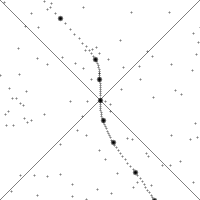2. 任何物体的速度不能超过光速：
翻译：静止的物体的世界线是平行与时间轴$y$轴的直线，方向为虚方向，选择其它速度的参考系其实就是在旋转时空的坐标，根据旋转的几何性质，虚方向永远为虚方向，曲线斜率绝对值永远大于1，即速度永远小于光速，又由于旋转没有周期，匀双曲角速度旋转坐标系可一直进行，因此物体在它自己看来，可以永远匀加速但还是无法达到光速。
3. 时空中的距离可分为类空、类时、类光三类：
翻译：两点之间的距离分为的实数、虚数、0三类是有明确物理意义的。实数距离代表两点是空间间隔，总能找到一个参考系把它们变换到空间轴x轴上，我们将这种方向叫类空的；虚数距离说明两点是时间间隔，总能找到一个参考系它们变换到时间轴y轴上，这种方向叫类时的；0距离说明两点之间是“类光”间隔，因为在任何参考系下通过斜率计算速度都是光速。通常的有质量物质的世界线永远都是类时的，无质量粒子（如光子）的世界线永远都是类光的，不存在类空世界线的粒子，它们若存在将会违反时空的因果结构。
4. 粒子的一段世界线的长度为该粒子经历的固有时，即自己感受到的时间流逝。
翻译：当粒子静止时，世界线与y轴重合，所以两点间世界线长度跟两点时间差相同，叫固有时。双曲旋转前后不改变图形长度，因此固有时（长度）不变，坐标时（y坐标值）改变。
5. 物体运动起来时会产生尺缩效应与钟慢效应：
翻译：通过双曲旋转，在新的坐标系下看坐标分量就不难看出，具体构造方法可以参考上面提到的可视化相对论的视频。
6. 同时的概念是相对的，不同参考系下对“同时发生”看法可以存在不一致：
翻译：旋转坐标系不可能只动一根轴，时间轴旋转了方向，空间轴那么自然也会动（牛顿时空观则不会动），在新的坐标系下等时面也变得倾斜，拥有同样时间坐标的两点在另一坐标系下当然不一定还拥有同样的时间坐标值。车库悖论、堵火车悖论等经典相对论佯谬均来源于此，细节参考上面提到的可视化相对论的视频。

## 三维闵氏空间中的“球”

### 双叶双曲面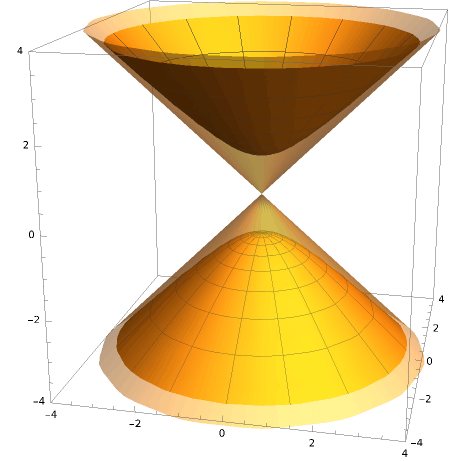$$x^2+y^2-z^2=-1$$这个方程是一张双叶双曲面。它可以看作由$xz$平面上的类空的双曲线绕$z$轴旋转而成的，双曲线的两条渐近线旋转后恰好得到圆锥形的光锥。这个双曲面还有什么几何性质呢？在闵氏空间最需要做的就是分辨方向类型。沿双曲面表面到底有哪些类型的方向呢？回顾一下，球面上的切向量一定垂直于半径方向，我们猜测双曲面上也是，只不过这里的“垂直”概念是新的闵氏空间中定义的垂直。两个向量垂直则它们的内积为0。欧氏空间中向量内积定义为$\langle(x_1,y_1,z_1),(x_2,y_2,z_2)\rangle=x_1x_2+y_1y_2+z_1z_2$，闵氏空间中它变成了$\langle(x_1,y_1,z_1),(x_2,y_2,z_2)\rangle=x_1x_2+y_1y_2-z_1z_2$。可以验证与类时向量垂直的向量一定是类空的，而双曲面上的所有切向量都垂直于“半径”方向。这些半径方向（从原点指向双曲面上的一点）都在光锥内，都是类时的虚方向，因此这张双曲面上的所有方向都类空，所有切向量的长度都是正的实数。

1. 根据两点之间直线最短，通过复杂的变分法可以计算得到，球面上两点(对径点例外)之间最短的曲线一定是大圆的劣弧。
2. 大圆是一种在球面上最“直”的曲线了，只是因为必须限制在曲面上才不得不随曲面弯曲。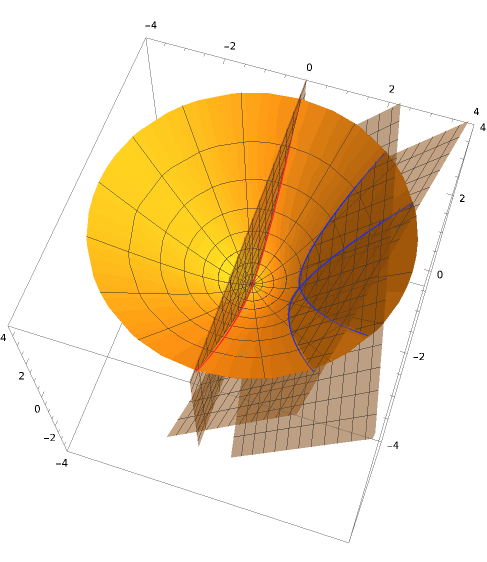### 单叶双曲面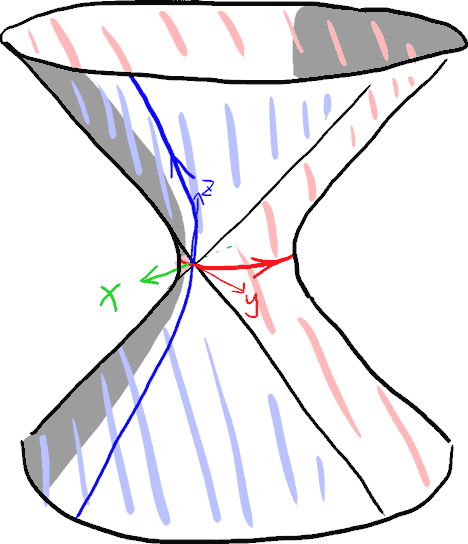$$x^2+y^2-z^2=1$$这个方程是一张单叶双曲面，与双叶双曲面的区别在等式右边的符号。它也可以看作由$xz$平面上的类时双曲线绕$z$轴旋转而成的。双曲线的两条渐近线旋转后也恰好得到圆锥形的光锥。单叶双曲面跟双叶双曲面的性质就不太一样了。上图中选定点$(1,0,0)$，我们可以往$y$、$z$两个方向走。显然，这两个方向一个类空一个类时，因此单叶双曲面上的空间仍然是一种时空，即它局部看起来像$\mathbb{R}^{1,1}$。不难想象，给出任意一点，我们还是可以在双曲面上做出过该点的光锥。其实它是一种常曲率的弯曲时空，这些东西对科学家研究广义相对论中宇宙整体结构有些帮助。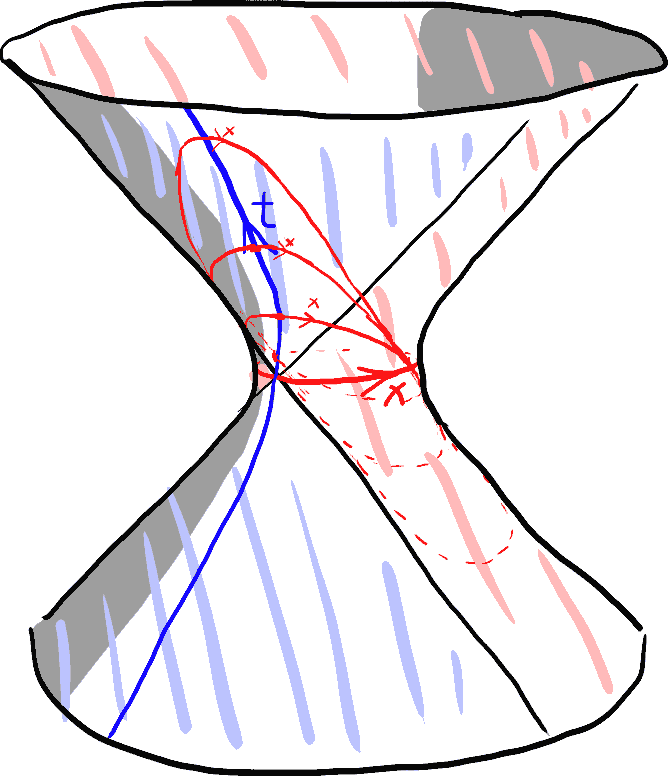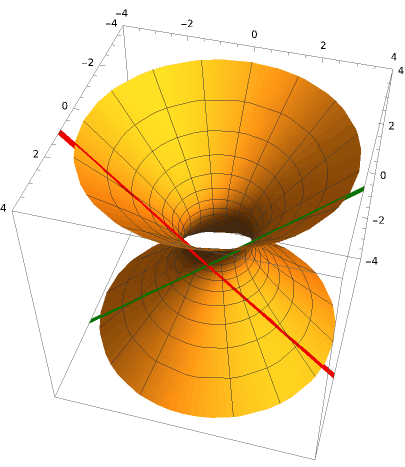### 德西特/反德西特时空

1. 德西特时空（de Sitter space）是四维常曲率时空，曲率为正，且宇宙是封闭的，空间体积有限；
2. 反德西特时空（Anti-de Sitter space）是四维常曲率时空，曲率为负，且宇宙在空间与时间上均无限；

#### 德西特时空

• 从五维闵氏空间到叶双曲面丢掉了一个间维度方向，于是剩下四个空间维；
• 从五维闵氏空间到叶双曲面丢掉了一个间维度方向，于是剩下三个空间维加一个时间维；

#### 反德西特时空

1. $$x^2+y^2+z^2-w^2-t^2=1$$先看这个双曲超曲面，根据平方的非负性可知，它跟所有空间轴（$x$、$y$、$z$轴）有交点，跟所有时间轴（$w$、$t$轴）无交点。拿$x$轴为例，交点附近的超曲面上的切空间方向应该有除了$x$方向外的所有方向，即有$y$、$z$两个空间方向和$w$、$t$两个时间方向。可见我们选错了，并不经意间得到了一种常曲率的二维空间+二维时间的怪异空间。
2. $$x^2+y^2+z^2-w^2-t^2=-1$$同理可以验证它确实是3+1维时空，看来这就是正确的选择了。令$t=0$，得到$x^2+y^2+z^2-w^2=-1$，它的空间形状是四维闵氏空间中的一个负常曲率的三维双曲空间，因此它确实是反德西特时空。

#### 二维反德西特时空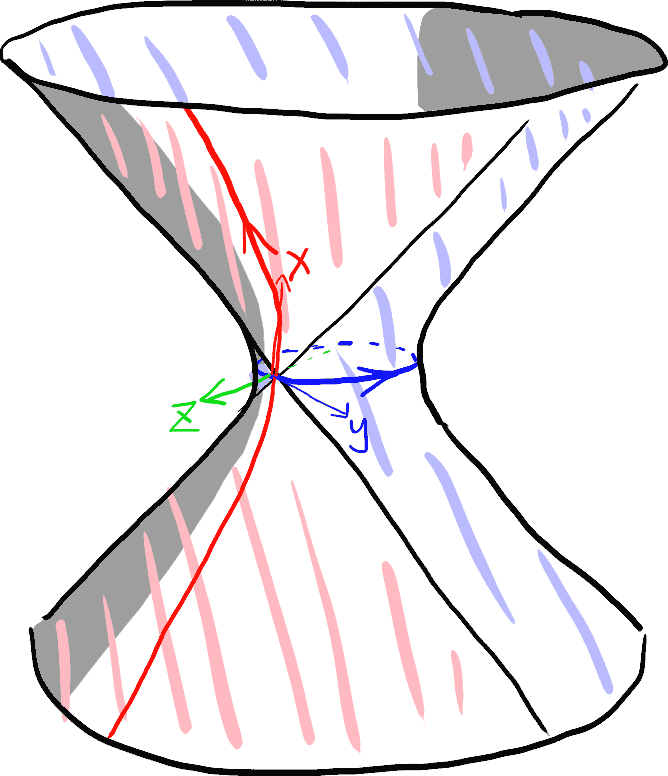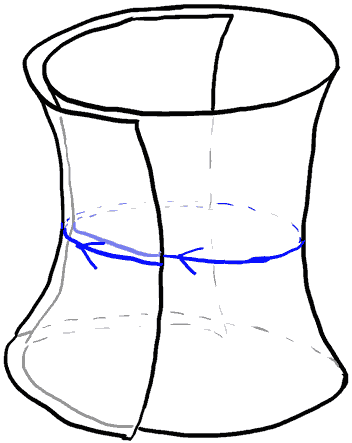## 内禀几何曲率简介

#### 黎曼曲率张量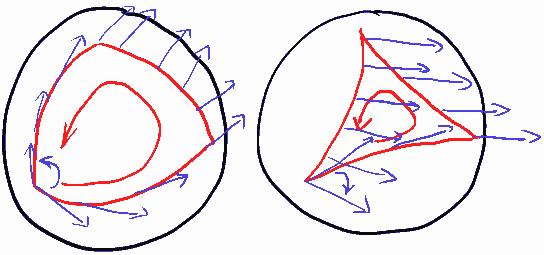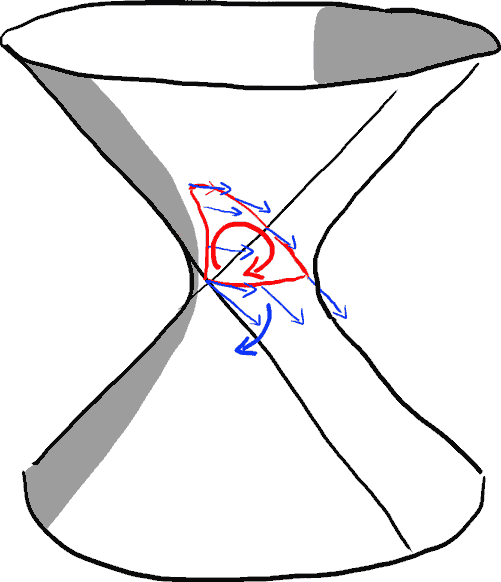1. 首先看路径部分：我们记$e_{xy}$为沿$x\rightarrow y\rightarrow -x\rightarrow -y$ 的走过的带方向的平行四边形。注意这个东西其实就是Blog上很早讲过的2-向量。如果不小心交换了平行四边形的两条边，代表的路径方向就会倒过来，即$e_{xy}=-e_{yx}$，注意无论$x$、$y$是什么方向这个等式都是成立的。
2. 接着看旋转部分：我们记$e_{x}^y$为从$x$到$y$方向的单位速度的旋转。这样写上下标代表这个旋转是输入$x$输出$y$的变换，代表着这个旋转下$x$轴上的点的速度方向是$y$轴。同理$e_{y}^x$为从$y$到$x$方向的单位速度的旋转。如果$x$与$y$方向的性质相同，则就是普通旋转，这两个旋转方向相反，有$e_{x}^y=-e_{y}^x$；如果$x$与$y$方向的性质不同，则其实是双曲旋转，这两个方向是相同的，有$e_{x}^y=e_{y}^x$！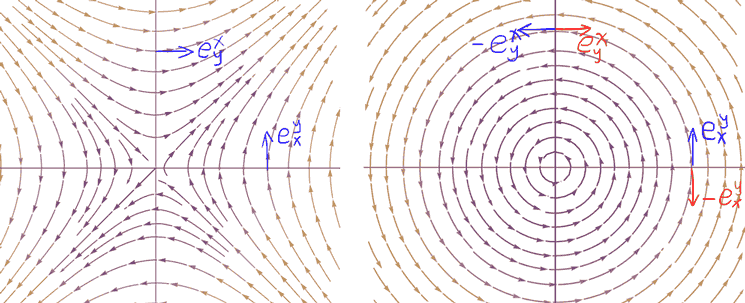把一个向量映射到另一个向量的映射可以自然看成把一个向量和一个对偶向量映射成数的映射，形象解释可参考脑洞《参观无限大养猪场》。根据对偶空间的相关理论，我们需要将上下标统一，要想将上标拉下来就必须用到空间的度量，具体体现在把上标拉下来时，类空向量不变，类时向量符号会反号。举两个例子：

1. 纯空间方向的旋转有$e_{x}^y=-e_{y}^x$，将指标全变下标则为$e_{xy}=-e_{yx}$，跟路径的那个反对称式子相同。
2. 设$x$类空，$y$类时，则双曲旋转满足$e_{x}^y=e_{y}^x$，将指标全变下标则为$-e_{xy}=e_{yx}$，还是跟路径的那个反对称式子相同。